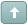Error

 Previous Topic Next Topic
 KTP2 #1 Posted : Tuesday, May 4, 2010 4:37:51 PM(UTC)Rank: Advanced MemberGroups: Registered, Registered Users, SubscribersJoined: 2/2/2007(UTC)Posts: 367Was thanked: 1 time(s) in 1 post(s) Regularization Chris Satchwell's article, "Regularization" included the calculation method for two indicators; a Regularized Exponential Moving Average and a Regularized Momentum. The MetaStock formulas below prompt for the number of periods in the moving average and for the regularization constant. Since the article did not include a range for the regularization constant, the formulas allows for any values between 0 and 20. To create a formula in MetaStock, select Indicator Builder from the Tools menu. Click New and then enter the formula below. When finished click OK. Repeat for the second formula. Name: Regularized EMA Formula: x:=Input("number of periods in moving average",1,500,21); d:=Input("regularization constant",0,20,.5); a:=2/(x+1); (PREV*(1+(2*d))+(a*(C-PREV))-(d*Ref(PREV,-1)))/(1+d) Name: Regularized Momentum Formula: x:=Input("number of periods in moving average",1,500,21); d:=Input("regularization constant",0,20,.5); a:=2/(x+1); f:=(PREV*(1+(2*d))+(a*(C-PREV))-(d*Ref(PREV,-1)))/(1+d); (f-Ref(f,-1))/fWanna join the discussion?! Login to your Discussions forum accountor Register a new forum account.Users browsing this topic
Forum Jump
You cannot post new topics in this forum.
You cannot reply to topics in this forum.
You cannot delete your posts in this forum.
You cannot edit your posts in this forum.
You cannot create polls in this forum.
You cannot vote in polls in this forum.